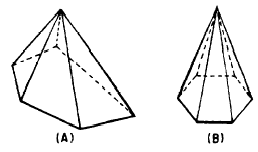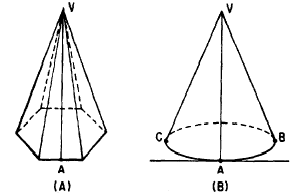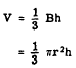Regular pyramids and right circular conesCustom SearchREGULAR PYRAMIDS AND RIGHT CIRCULAR CONES A PYRAMID is a solid figure, the lateral faces of which are triangles. (See fig. 18-16.) A REGULAR PYRAMID has all of its lateral faces equal.Figure 18-16.-(A) Irregular pyramid; (B) regular pyramid. A regular pyramid with a very large number of lateral faces would have a base polygon with many sides. If the number of sides is sufficiently large, the base polygon is indistinguishable from a circle and the surface formed by the many lateral faces becomes a smoothly curved surface. The solid figure thus formed is a RIGHT CIRCULAR CONE. (See fig. 18-17.)Figure 18-17. -Right circular cone. Slant Height The slant height of a regular pyramid is the perpendicular distance from the vertex to the center of any side of the base. For example, the length of line AV in figure 18-18 (A) is the slant height. The slant height of a right circu base. Such a line is perpendicular to a line tangent to the base at the point where the slant height intersects the base. (See fig. 18-18 (B).) Lines AV, BV, and CV in figure 18-18 (B) are all slant heights.Figure 18-18.-(A) Slant height of a regular pyramid; (B) slant height of a right circular cone. Lateral Area The lateral area of a pyramid is the sum of the areas of its lateral faces. If the pyramid is regular, its lateral faces have equal bases; furthermore, the slant height is the altitude of each face. Therefore, the area of each lateral face is one-half the slant height multiplied by the length of one side of the base polygon. Since the sum of these sides is the perimeter of the base, the total lateral area of the pyramid is the product of one-half its slant height multiplied by the perimeter of its base. Using s to represent slant height and P to represent the perimeter of the base, the formula is as follows: Lateral Area = 1/2 aP A right circular cone can be considered as a regular pyramid with an infinite number of faces. Therefore, using C to represent the circumference of the base, the formula for the lateral area of a right circular cone is Lateral Area = 1/2 sC Volume The volume of a pyramid is determined by its base and its altitude, as is the case with other solid figures. Experiments show that the volume of any pyramid is-one-third of the prod stated as a formula with V representing volume, B representing the area of the base, and h representing height (altitude), as follows: V = 1/3 Bh The formula for the volume of a pyramid does not depend in any way upon the number of faces. Therefore, we use the same formula for the volume of a right circular cone. Since the base is a circle, we replace B with pr2 (where r is the radius of the base). The formula for the volume of a right circular cone is thenPractice problems: 1. Find the lateral area of a regular pyramid with a 5-sided base measuring 3 inches on each side, if the slant height is 12 inches. 2. Find the lateral area of a right circular cone whose base has a diameter of 6 cm and whose slant height is 14 cm. 3. Find the volume of a regular pyramid with a square base measuring 4 cm on each side, if the vertex is 9 cm above the base. 4. Find the volume of a right circular cone whose base has a diameter of 14 inches, if the altitude is 21 inches. Answers: 1. 90 in. sq  2. 132 cm sq  3. 48 cu cm 4. 1077 cu in.Integrated Publishing, Inc. - A (SDVOSB) Service Disabled Veteran Owned Small Business